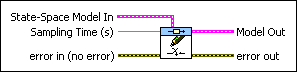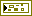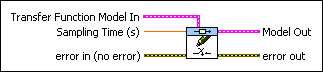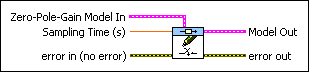# CD Set Sampling Time to Model VI

LabVIEW 2018 Control Design and Simulation Module Help

Edition Date: March 2018

Part Number: 371894J-01

»View Product InfoDownload Help (Windows Only)

Owning Palette: Model Information VIs

Requires: Control Design and Simulation Module

Sets the sampling time of the system. Wire data to the State-Space Model In input to determine the polymorphic instance to use or manually select the instance.

Use the pull-down menu to select an instance of this VI.

 Select an instance CD Set Sampling Time to Model (State-Space)CD Set Sampling Time to Model (Transfer Function)CD Set Sampling Time to Model (Zero-Pole-Gain)

## CD Set Sampling Time to Model (State-Space)State-Space Model In contains a mathematical representation of and information about the system for which this VI sets sampling time.Sampling Time (s) specifies the sampling time of the model. Sampling Time (s) defines whether the model represents a continuous-time system or a discrete-time system. If the model represents a continuous-time system, Sampling Time (s) must equal 0. If the model represents a discrete-time system, Sampling Time (s) must be greater than 0 and equal to the sampling rate, in seconds, of the discrete system. A value of -1 specifies that Sampling Time (s) is irrelevant. The default is 0.error in describes error conditions that occur before this node runs. This input provides standard error in functionality.Model Out is the modified system model with a new sampling time. To access and modify the data in the model, use the Model Information VIs.error out contains error information. This output provides standard error out functionality.

## CD Set Sampling Time to Model (Transfer Function)Transfer Function Model In contains a mathematical representation of and information about the system for which this VI sets sampling time.Sampling Time (s) specifies the sampling time of the model. Sampling Time (s) defines whether the model represents a continuous-time system or a discrete-time system. If the model represents a continuous-time system, Sampling Time (s) must equal 0. If the model represents a discrete-time system, Sampling Time (s) must be greater than 0 and equal to the sampling rate, in seconds, of the discrete system. A value of -1 specifies that Sampling Time (s) is irrelevant. The default is 0.error in describes error conditions that occur before this node runs. This input provides standard error in functionality.Model Out is the modified system model with a new sampling time. To access and modify the data in the model, use the Model Information VIs.error out contains error information. This output provides standard error out functionality.

## CD Set Sampling Time to Model (Zero-Pole-Gain)Zero-Pole-Gain Model In contains a mathematical representation of and information about the system for which this VI sets sampling time.Sampling Time (s) specifies the sampling time of the model. Sampling Time (s) defines whether the model represents a continuous-time system or a discrete-time system. If the model represents a continuous-time system, Sampling Time (s) must equal 0. If the model represents a discrete-time system, Sampling Time (s) must be greater than 0 and equal to the sampling rate, in seconds, of the discrete system. A value of -1 specifies that Sampling Time (s) is irrelevant. The default is 0.error in describes error conditions that occur before this node runs. This input provides standard error in functionality.Model Out is the modified system model with a new sampling time. To access and modify the data in the model, use the Model Information VIs.error out contains error information. This output provides standard error out functionality.